# MA.6.NSO.4.2Export Print
Apply and extend previous understandings of operations with whole numbers to multiply and divide integers with procedural fluency.

### Clarifications

Clarification 1: Instruction includes the use of models and number lines and the inverse relationship between multiplication and division, working towards becoming procedurally fluent by the end of grade 6.

Clarification 2: Instruction focuses on the understanding that integers can be divided, provided that the divisor is not zero, and every quotient of integers (with non-zero divisor) is a rational number. If p and q are integers where q≠0, then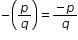,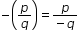and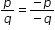.

General Information
Subject Area: Mathematics (B.E.S.T.)
Strand: Number Sense and Operations
Status: State Board Approved

## Benchmark Instructional Guide

• Dividend
• Divisor
• Expression
• Factor
• Integer
• Number Line

### Vertical Alignment

Previous Benchmarks

http://flbt5.floridaearlylearning.com/standards.html

Next Benchmarks

### Purpose and Instructional Strategies

Prior to grade 6, students have multiplied whole numbers and positive rational numbers. This is their first experience multiplying and dividing with negative numbers. In grade 6, students will use these skills to write and solve one step equations. Students will continue to develop these skills in grade 7, with multiplying and dividing rational numbers in all forms and using them to solve equations and inequalities.
• Instruction includes extending the understanding that a negative integer is the same distance from zero, but the opposite direction to multiplying and dividing integers.
• For example, −6 · 4 can be thought of as “the opposite of 6 times 4.” Since 6 times 4 is 24, the opposite of 24 is –24.
• Instruction includes using algebra tiles or two-color counters to model multiplication and division of integers. Students flip the tiles over as directed by the negative value being “the opposite of.”
• Algebra Tiles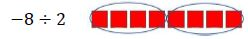• Two-Color Counters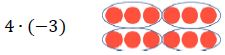• Use guided discussion for students to notice and record patterns. From analyzing the pattern, students should conclude that when two positive or two negative integers are multiplied or divided, the resulting product or quotient is positive. If a negative and a positive number are multiplied or divided, the resulting product or quotient is negative (MTR.2.1, MTR.5.1).
• Dividing a negative by a negative can be interpreted as was done when dividing a whole number by a whole number.
• For example, −8 divided by −4 can be interpreted as “How many groups of -4 are in −8,” or as “What is the opposite of the size of the group that you get if you divide a group of −8 into 4 parts?”.
• Division should be shown using the ÷ symbol when written horizontally and a fraction bar when written vertically.
• If an integer divided by an integer results in a rational number quotient, the quotient can be written as a fraction or as a decimal (MTR.3.1).
• Multiplication and division with integers are inverse relationships resulting in related equations (MTR.3.1, MTR.5.1).
• Students should develop fluency with and without the use of a calculator when performing operations with integers.
• Instruction includes rewriting negative fractions using the division symbol and grouping symbols.
• The teacher can refer to the negative sign as “the opposite of” to represent the entire quotient is the opposite of its positive value, which will be negative.
• For example, −$\frac{\text{50}}{\text{10}}$  = −(50 ÷ 10) which is equivalent to −(5), which is equivalent to −5.
• The teacher can refer to the negative sign as multiplying by −1.
• For example, −$\frac{\text{50}}{\text{10}}$ = −1($\frac{\text{50}}{\text{10}}$) which is equivalent to − 1(50 ÷ 10), which is equivalent to −1(5), which is equivalent to −5.
• The teacher can make connections to the properties of operations (Appendix D).
• For example, −$\frac{\text{50}}{\text{10}}$ = −1($\frac{\text{50}}{\text{10}}$) which is equivalent to − 1(50 ÷ 10), which is equivalent to (−50) ÷ 10, which is equivalent to −5.
• For example, −$\frac{\text{50}}{\text{10}}$ = −1 ($\frac{\text{50}}{\text{10}}$) which is equivalent to − 1(50 ÷ 10), which is equivalent to 50 ÷ (−10), which is equivalent to −5.

### Common Misconceptions or Errors

• Students may incorrectly apply rules to multiplication and division of integers without experiencing models to provide concrete understanding. Using algebra tiles or two-colored counters, have students flip over the tiles every time they encounter a negative. This allows students to see a pattern and see negatives as “the opposite of” (MTR.2.1, MTR.5.1).
• Students may incorrectly believe the sign of the product or quotient is determined by the integer that is further from zero because they are confusing the pattern or rules for adding and subtracting integers with multiplying and dividing integers.
• Students may incorrectly conclude if an expression begins with a positive integer, the value of the expression is positive or if it begins with a negative integer, the value of the expression is negative.
• Students may incorrectly assume if division is written as a negative fraction, both the numerator and denominator are negative values. This can be addressed by modeling with algebra tiles or colored counters.
• Students may confuse zero for being a negative number because it is less than a whole number. However, 0 is a neutral number; it is neither positive nor negative.
• Students may say that negative value divided by itself is zero because they subtract instead of divide. Students should remember that any number divided by itself is equal to 1.
• Students may make the common mistake of dividing the numbers but not remembering to pay attention to the negative sign. It may help students to write or highlight their negative signs in color to make them stand out visually.

### Strategies to Support Tiered Instruction

• Teacher provides opportunities for students to utilize two-color counters to represent multiplication as repeated addition to develop generalizations for multiplying integers and discuss the difference between generalized rules for addition and generalized rules for multiplication.
• Teacher provides opportunities for students to utilize two-color counters when evaluating the product or quotient of integers. Instruction includes having students write the expression, representing the expression with the two-color counters, finding the product or quotient, and then recording the solution.
• Teacher provides instruction on the connections between dividends and divisors and numerators and denominators.
• Teacher provides algebra tiles or two-colored counters, to have students flip over the tiles every time they encounter a negative. This allows students to see a pattern and see negatives as “the opposite of.”
• Teacher models with algebra tiles or colored counters.
• Teacher models how to write or highlight their negative signs in color to make them stand out visually.

Camden is trying to understand why the product of a positive number and a negative number should be negative. How would you explain to Camden why 5(−4) is a negative number

Shannon noticed that – 2[5 + (−3)] can be rewritten using the Distributive Property as:

−2 · 5 + (−2) · (−3)

= −10 + ______

= −4

She was not sure what to put in the blank.
• Part A. What number must go in the blank so the answer is −4?
• Part B. What does this tell Shannon about the result of multiplying two negative numbers?

MTR.7.1)
Describe a real-world problem that can be solved by dividing −12 by 3. Then, find the quotient and explain what it means in the context of your problem.

### Instructional Items

Instructional Item 1
Model one method for evaluating –4×5.

Instructional Item 2
What is the value of the expression –72 ÷ 8?

Instructional Item 3
Select all of the values that are equivalent.
a. $\frac{\text{1}}{\text{−4}}$
b. −$\frac{\text{20}}{\text{5}}$
c. 4

d. − ($\frac{\text{−5}}{\text{−20}}$)

e. −$\frac{\text{1}}{\text{4}}$
f. $\frac{\text{−5}}{\text{−20}}$

*The strategies, tasks and items included in the B1G-M are examples and should not be considered comprehensive.

## Related Courses

This benchmark is part of these courses.
1200400: Foundational Skills in Mathematics 9-12 (Specifically in versions: 2014 - 2015, 2015 - 2022, 2022 and beyond (current))
1205010: M/J Grade 6 Mathematics (Specifically in versions: 2014 - 2015, 2015 - 2022, 2022 and beyond (current))
1205020: M/J Accelerated Mathematics Grade 6 (Specifically in versions: 2014 - 2015, 2015 - 2020, 2020 - 2022, 2022 and beyond (current))
1204000: M/J Foundational Skills in Mathematics 6-8 (Specifically in versions: 2014 - 2015, 2015 - 2022, 2022 and beyond (current))
7812015: Access M/J Grade 6 Mathematics (Specifically in versions: 2014 - 2015, 2015 - 2018, 2018 - 2022, 2022 and beyond (current))

## Related Access Points

Alternate version of this benchmark for students with significant cognitive disabilities.
MA.6.NSO.4.AP.2: Use tools to multiply and divide integers between 20 and −20.

## Related Resources

Vetted resources educators can use to teach the concepts and skills in this benchmark.

## Educational Software / Tool

Arithmetic Quiz:

In this activity, students solve arithmetic problems involving whole numbers, integers, addition, subtraction, multiplication, and division. This activity allows students to track their progress in learning how to perform arithmetic on whole numbers and integers. This activity includes supplemental materials, including background information about the topics covered, a description of how to use the application, and exploration questions for use with the java applet.

Type: Educational Software / Tool

## Formative Assessments

Negative Times:

Students are shown a problem that illustrates why the product of two negatives is a positive and are asked to provide a rationale.

Type: Formative Assessment

Integer Division:

Students are asked to describe a real-world context for a given expression involving the quotient of two rational integers.

Type: Formative Assessment

## Lesson Plans

Have You Met Your Match?-Understanding Equivalent Expressions :

In this lesson, students will use the properties of operations to generate and identify equivalent algebraic expressions. Students will apply their prior knowledge of the distributive property and combining like terms to create equivalent algebraic expressions. The hands-on memory "match" game will engage students and support student understanding of algebraic thinking.

Type: Lesson Plan

Order Matters:

Students will analyze a Scratch program and compare its computerized algorithm to the mathematical order of operations, in this lesson plan.

Type: Lesson Plan

## Original Student Tutorial

Why Does a Negative Times a Negative Equal a Positive?:

Use mathematical properties to explain why a negative factor times a negative factor equals a positive product instead of just quoting a rule with this interactive tutorial.

Type: Original Student Tutorial

## MFAS Formative Assessments

Integer Division:

Students are asked to describe a real-world context for a given expression involving the quotient of two rational integers.

Negative Times:

Students are shown a problem that illustrates why the product of two negatives is a positive and are asked to provide a rationale.

## Original Student Tutorials Mathematics - Grades 6-8

Why Does a Negative Times a Negative Equal a Positive?:

Use mathematical properties to explain why a negative factor times a negative factor equals a positive product instead of just quoting a rule with this interactive tutorial.

## Student Resources

Vetted resources students can use to learn the concepts and skills in this benchmark.

## Original Student Tutorial

Why Does a Negative Times a Negative Equal a Positive?:

Use mathematical properties to explain why a negative factor times a negative factor equals a positive product instead of just quoting a rule with this interactive tutorial.

Type: Original Student Tutorial

## Educational Software / Tool

Arithmetic Quiz:

In this activity, students solve arithmetic problems involving whole numbers, integers, addition, subtraction, multiplication, and division. This activity allows students to track their progress in learning how to perform arithmetic on whole numbers and integers. This activity includes supplemental materials, including background information about the topics covered, a description of how to use the application, and exploration questions for use with the java applet.

Type: Educational Software / Tool

## Parent Resources

Vetted resources caregivers can use to help students learn the concepts and skills in this benchmark.